People love rankings. You've probably seen articles about the best places to live, the best colleges to attend, the best pizza to order, and so on. Each of these is an example of a ranking that is based on multiple characteristics. For example, a list of the best places to live might base the ranking on quantities such as the average salary, the cost of homes, crime rates, quality of schools, and so forth. Pizzas might be ranked on the crust, the sauce, the toppings, and more. Typically, the ranking is a weighted average of these multiple variables.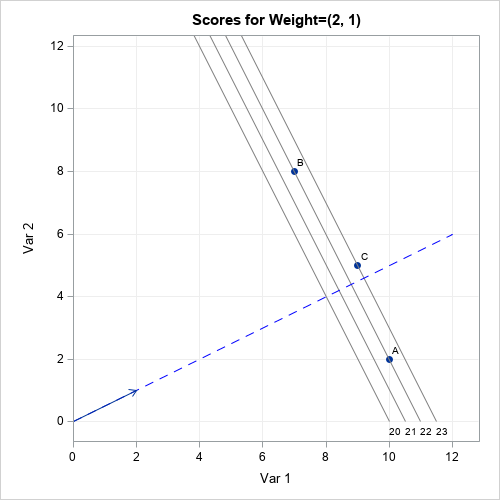Inevitably, after the rankings are released, some people complain that the weights used to construct the ranking were not fair. The most common complaint is that the weights were chosen arbitrarily by the editors. This is a valid criticism: someone must choose the weights, but not everyone will agree with the choice. Some organizations base the weights on surveys in which people are asked to weigh the relative importance of the factors. Other organizations might use equal weights for the characteristics. Others just make up weights that seem to work well.

This article discusses the geometry of a weighted mean (or sum) of multiple variables. When you specify the weights, you are choosing a direction (a line) in the space of the variables. Geometrically, each subject (city, college, pizza, ...) is projected onto the line. The ranking is then determined by the relative placement of each subject when projected onto the line. This is shown visually by the graph to the right. The details of the graph are explained later in this article.

Mathematically, a weighted mean and a weighted sum are very similar and result in the same ranks. I will use the weighted sum in this article. If you prefer, you can get the weighted mean by dividing the weighted sum by the number of factors. Also, a projection onto a line requires scaling the weight vector to have unit length, but I will ignore that technical detail.

### Two-dimensional rankings

To illustrate the geometry of ranking subjects, consider the case where there are only two characteristics. Suppose three students are being evaluated for admission to a university program. Each subject submits two scores on a standardized test. The following table shows the test scores for the three students: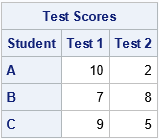Which student is the best fit for the university program? It depends on how the program weights the two test scores. Let's pretend that "Test 1" is a test of mathematical and analytical skills and that "Test 2" is a test of verbal and language skills. Some programs (math, engineering, and other STEM disciplines) will put extra weight on the math score, whereas other programs (creative writing, linguistics, journalism, ...) will put extras weight on the verbal score. Here are a few ways that various programs might weigh the tests:

• A math department might look only at the math test and ignore the language test. This corresponds to the weight vector w1=(1,0).
• A law school might want to equally weigh the math and language tests. This corresponds to the weight vector w2=(1,1).
• An engineering program might decide that math skills are twice as important as language skills. This corresponds to the weight vector w3=(2,1).

The following table displays the weighted sums for each student under these different choices for weights: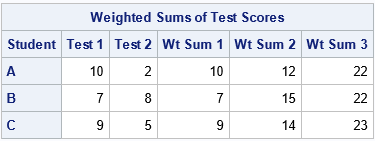The table shows that the "best" candidate depends on the weights that the programs choose:

• For the math department ("Wt Sum 1"), Student A is the best candidate.
• For the law school ("Wt Sum 2"), Student B is the best candidate.
• For the engineering program ("Wt Sum 3"), Student C is the best candidate.

### The geometry of weighted averages

You can plot the test scores as ordered pairs in two-dimensional space. The weight vector determines a line. The projection of the ordered pairs onto that line determines the ranking of the subjects. For example, the following graph shows the geometry for the weight vector w1=(1,0):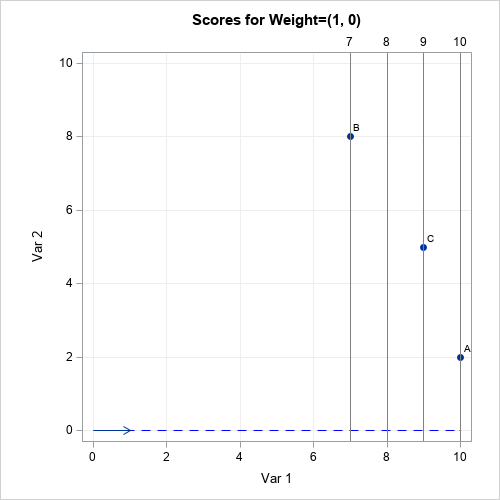For this weight vector, the weighted sum is merely the first component. This is also the projection of the point onto the blue dashed line αw1, for any scalar value of α. The projections that are far from the origin have a larger weighted sum than the projections that are closer to the origin. The students' ranks are determined by their relative positions along the blue dashed line. For this example, Student A is the most highly ranked student (for the math department) because its projection onto the line is farthest from the origin. The gray lines indicate the projection onto the blue line. The gray lines are perpendicular to the blue line.

If you use a different weight vector, you might get a different result. The following graph shows the results for the same students but for the law-school weight vector w2=(1,1). Again, the students' ranks are determined by their relative positions along the blue dashed line. But this time, Student B is the most highly ranked student because its projection onto the line is farthest from the origin. The gray lines are perpendicular to the blue line and indicate the projection onto the blue line.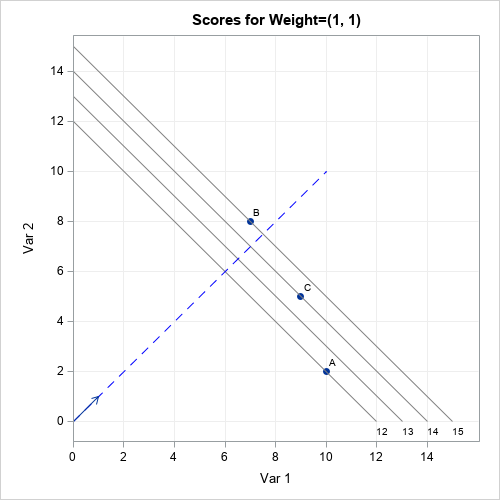The ranks of the students change again if you consider the weights given by w3=(2,1). For these weights, Student C is the highest-ranked student by the engineering school. The graph is shown at the top of this article. Note that students A and B are assigned the same rank because they lie on the same gray line, which is perpendicular to the blue line. This can happen for any weight vector: If two students are on the same perpendicular line, their projection is the same.

### Ranking by using additional variables

The geometry does not change much if you compute a weighted sum of more than two factors. For three factors, the weight vector determines a (blue dashed) line in 3-D space, and the scores are projected onto the line. In the 2-D examples, I drew gray lines perpendicular to the blue line. For three factors, the analogous structures are planes that are perpendicular to the blue line. All subjects on the same plane have the same ranking. In d dimensions, the planes become (d-1)-dimensional hyperplanes.

An important consideration for this process (in any dimension) is that the factors should be measured on comparable scales. In the earlier example, both standardized test scores were assumed to be measured on the same scale. However, you should standardize variables that are on different scales. For example, you would not want to use a weighted average of the median home price (possibly \$300,000) and the mean annual temperature (possibly 15°C) without standardizing those quantities.

### Summary

Sometimes rankings are based on a statistical model, but other times they are based on a "gut feeling" about which features are important. Magazines publish annual rankings of colleges, cities, hospitals, pizzas, and places to work. It is important to realize that the weights that are used for these rankings are somewhat arbitrary. Even if the weights are based on a survey, there is uncertainty in the weights.

This article shows that if you vary the weights, the rankings can change. This article discusses the geometry of weighted sums and averages. A weight vector determines a direction in a d-dimensional feature space. To rank subjects, you project d-dimensional tuples of points onto the line that is determined by the weight vector. The highest-ranked subject is the one that is farthest from the origin along the direction of the weight vector.

So next time you disagree with a ranking, you can confidently say to your friends, "Yes, but if they had used different weights, the results would have been different."

The post Rankings and the geometry of weighted averages appeared first on The DO Loop.This article uses simulation to demonstrate the fact that any continuous distribution can be transformed into the uniform distribution on (0,1). The function that performs this transformation is a familiar one: it is the cumulative distribution function (CDF). A continuous CDF is defined as an integral, so the transformation is called the probability integral transformation.

This article demonstrates the probability integral transformation and its close relative, the inverse CDF transformation. These transformations are useful in many applications, such as constructing statistical tests and simulating data.

### The probability integral transform

Let X be a continuous random variable whose probability density function is f. Then the corresponding cumulative distribution function (CDF) is the integral $F(x) = \int\nolimits_{-\infty}^{x} f(t)\,dt$. You can prove that the random variable Y = F(X) is uniformly distributed on (0,1). In terms of data, if {X1, X2, ..., Xn} is a random sample from X, the values {F(X1), F(X2), ..., F(Xn)} are a random sample from the uniform distribution.

Let's look at an example. The following SAS DATA step generates a random sample from the Gamma distribution with shape parameter α=4. At the same time, the DATA step computes U=F(X), where F is the CDF of the gamma distribution. A histogram of the U variable shows that it is uniformly distributed:

/* The probability integral transformation: If X is a random variable with CDF F, then Y=F(X) is uniformly distributed on (0,1). */ %let N = 10000; data Gamma4(drop=i); call streaminit(1234); do i = 1 to &N; x = rand("Gamma", 4); /* X ~ Gamma(4) */ u = cdf("Gamma", x, 4); /* U = F(X) ~ U(0,1) */ output; end; run;   title "U ~ U(0,1)"; proc sgplot data=Gamma4; histogram U / binwidth=0.04 binstart=0.02; /* center first bin at h/2 */ yaxis grid; run;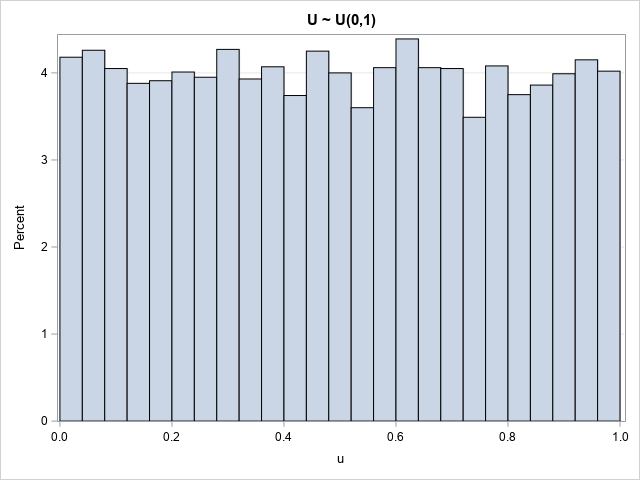The histogram has some bars that are greater than the average and others that are less than the average. That is expected in a random sample.

One application of the CDF transformation is to test whether a random sample comes from a particular distribution. You can transform the sample by using the CDF function, then test whether the transformed values are distributed uniformly at random. If the transformed variates are uniformly distributed, then the original data was distributed according to the distribution F.

### The inverse probability transformation

It is also useful to run the CDF transform in reverse. That is, start with a random uniform sample and apply the inverse-CDF function (the quantile function) to generate random variates from the specified distribution. This "inverse CDF method" is a standard technique for generating random samples.

The following example shows how it works. The DATA step generates random uniform variates on (0,1). The variates are transformed by using the quantiles of the lognormal(0.5, 0.8) distribution. A call to PROC UNIVARIATE overlays the density curve of the lognormal(0.5, 0.8) distribution on the histogram of the simulated data. The curve matches the data well:

/* inverse probability transformation */ data LN(drop=i); call streaminit(12345); do i = 1 to &N; u = rand("Uniform"); /* U ~ U(0,1) */ x = quantile("Lognormal", u, 0.5, 0.8); /* X ~ LogNormal(0.5, 0.8) */ output; end; run;   proc univariate data=LN; var x; histogram x / lognormal(scale=0.5 shape=0.8) odstitle="X ~ LogNormal(0.5, 0.8)"; run;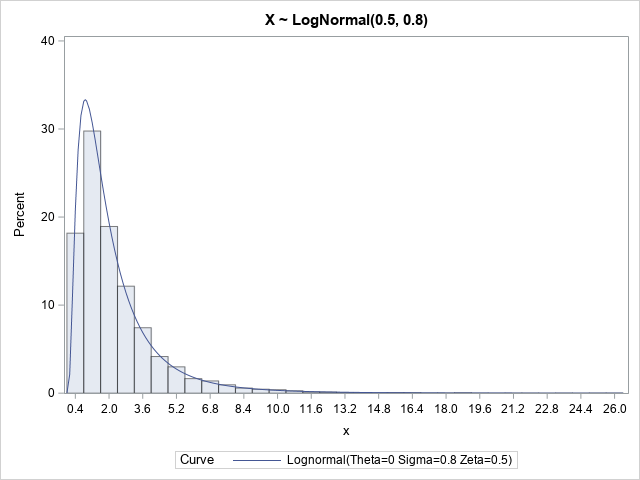### Summary

The probability integral transform (also called the CDF transform) is a way to transform a random sample from any distribution into the uniform distribution on (0,1). The inverse CDF transform transforms uniform data into a specified distribution. These transformations are used in testing distributions and in generating simulated data.

### Appendix: Histograms of uniform variables

The observant reader might have noticed that I used the BINWIDTH= and BINSTART= options to set the endpoints of the bin for the histogram of uniform data on (0,1). Why? Because by default the histogram centers bins. For data that are bounded on an interval, you can use the BINWIDTH= and BINSTART= options to improve the visualization.

Let's use the default binning algorithm to create a histogram of the uniform data:

title "U ~ U(0,1)"; title2 "34 Bins: First Bin Centered at 0 (Too Short)"; proc sgplot data=Gamma4; histogram u; yaxis grid; run;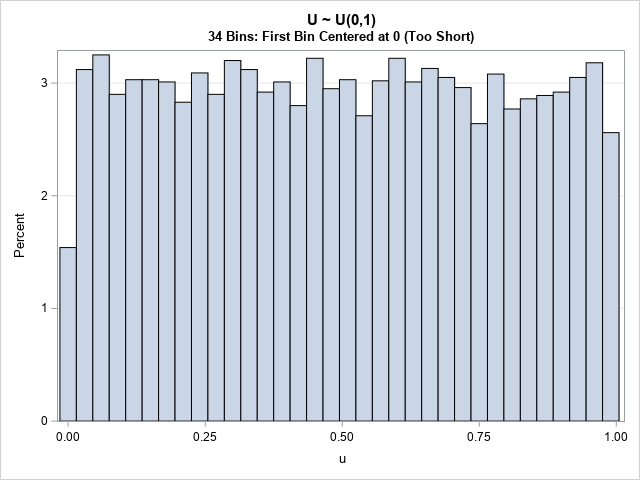Notice that the first bin is "too short." The first bin is centered at the location U=0. Because the data cannot be less than 0, the first bin has only half as many counts as the other bins. The last bin is also affected, although the effect is not usually as dramatic.

The histogram that uses the default binning is not wrong, but it doesn't show the uniformity of the data as clearly as a histogram that uses the BINWIDTH= and BINSTART= options to force 0 and 1 to be the endpoints of the bins.

The post The probability integral transform appeared first on The DO Loop.The skewness of a distribution indicates whether a distribution is symmetric or not. The Wikipedia article about skewness discusses two common definitions for the sample skewness, including the definition used by SAS. In the middle of the article, you will discover the following sentence: In general, the [estimators]are both biased estimators of the population skewness. The article goes on to say that the estimators are not biased for symmetric distributions. Similar statements are true for the sample kurtosis.

This statement might initially surprise you. After all, the statistics that we use to estimate the mean and standard deviation are unbiased. Although biased estimates are not inherently "bad," it is useful to get an intuitive feel for how biased an estimator might be.

Let's demonstrate the bias in the skewness statistic by running a Monte Carlo simulation. Choose an unsymmetric univariate distribution for which the population skewness is known. For example, the exponential distribution has skewness equal to 2. Then do the following:

1. Choose a sample size N. Generate B random samples from the chosen distribution.
2. Compute the sample skewness for each sample.
3. Compute the average of the skewness values, which is the Monte Carlo estimate of the sample skewness. The difference between the Monte Carlo estimate and the parameter value is an estimate of the bias of the statistic.

In this article, I will generate B=10,000 random samples of size N=100 from the exponential distribution. The simulation shows that the expected value of the skewness is NOT close to the population parameter. Hence, the skewness statistic is biased.

### A Monte Carlo simulation of skewness

The following DATA step simulates B random samples of size N from the exponential distribution. The call to PROC MEANS computes the sample skewness for each sample. The call to PROC SGPLOT displays the approximate sampling distribution of the skewness. The graph overlays a vertical reference line at 2, which is the skewness parameter for the exponential distribution, and also overlays a reference line at the Monte Carlo estimate of the expected value.

%let NumSamples = 10000; %let N = 100; /* 1. Simulate B random samples from exponential distribution */ data Exp; call streaminit(1); do SampleID = 1 to &NumSamples; do i = 1 to &N; x = rand("Expo"); output; end; end; run;   /* 2. Estimate skewness (and other stats) for each sample */ proc means data=Exp noprint; by SampleID; var x; output out=MCEst mean=Mean stddev=StdDev skew=Skewness kurt=Kurtosis; run;   /* 3. Graph the sampling distribution and overlay parameter value */ title "Monte Carlo Distribution of Sample Skewness"; title2 "N = &N; B = &NumSamples"; proc sgplot data=MCEst; histogram Skewness; refline 2 / axis=x lineattrs=(thickness=3 color=DarkRed) labelattrs=(color=DarkRed) label="Parameter"; refline 1.818 / axis=x lineattrs=(thickness=3 color=DarkBlue) label="Monte Carlo Estimate" labelattrs=(color=DarkBlue) labelloc=inside ; run;   /* 4. Display the Monte Carlo estimate of the statistics */ proc means data=MCEst ndec=3 mean stddev; var Mean StdDev Skewness Kurtosis; run;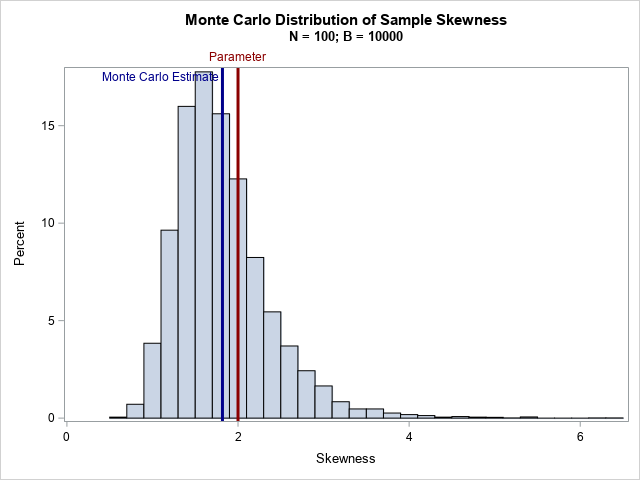For the exponential distribution, the skewness parameter has the value 2. However, according to the Monte Carlo simulation, the expected value of the sample skewness is about 1.82 for these samples of size 100. Thus, the bias is approximately 0.18, which is about 9% of the true value.

The kurtosis statistic is also biased. The output from PROC MEANS includes the Monte Carlo estimates for the expected value of the sample mean, standard deviation, skewness, and (excess) kurtosis. For the exponential distribution, the parameter values are 1, 1, 2, and 6, respectively. The Monte Carlo estimates for the sample mean and standard deviation are close to the parameter values because these are unbiased estimators. However, the estimates for the skewness and kurtosis are biased towards zero.

### Summary

This article uses Monte Carlo simulation to demonstrate bias in the commonly used definitions of skewness and kurtosis. For skewed distributions, the expected value of the sample skewness is biased towards zero. The bias is greater for highly skewed distributions. The skewness statistic for a symmetric distribution is unbiased.

The post The sample skewness is a biased statistic appeared first on The DO Loop.Testing people for coronavirus is a public health measure that reduces the spread of coronavirus. Dr. Anthony Fauci, a US infectious disease expert, recently mentioned the concept of "pool testing." The verb "to pool" means "to combine from different sources." In a USA Today article, Dr. Deborah Birx, the coordinator for the White House coronavirus task force, suggests that pooling can increase the number of people who get tested tenfold. What is pool testing? How does it enable you to test more people while running fewer tests? This article looks at the mathematics and statistics of pool testing.

### What is pool testing?

Testing reduces the spread of coronavirus. As of 23Jun2020, the US tests about 500,000 people per day, according to an article in the MIT Technology Review. Tests are given sick or exposed people to see if they should quarantine themselves and alert others. Tests are given to health-care professionals and other front-line workers on a regular basis to ensure that these workers are healthy and can continue to serve the public. In short, it is important to be able to process massive numbers of tests every day. Pool testing can test more people with the same number of tests.

Pool testing is not a new idea. It has been used for more than a decade to ensure the safety of donated blood. When people donate blood, the blood bank tests the blood to make sure it does not contain viruses such as HIV, hepatitis, West Nile, or Zika. As the name implies, pool testing involves taking a portion of blood from multiple individuals and combining the portions into a pooled sample. (Reserve some blood from each individual in case you need a second test.) The viral test (usually nucleic acid testing, or NAT) is run on the pooled sample. If the test is negative, you conclude that all of the individual samples are negative. If the test is positive, you know that one or more of the individual samples is positive. You do not know which samples are positive, but that's okay because you reserved a portion of each sample. You can run a second round of tests on the reserved samples, one at a time, to discover which individual or individuals are infected.

There are several kinds of COVID-19 tests, but one type uses NAT on nasal secretions from a donor. I am a statistician, not a medical expert, but I assume that pooling nasal secretions are similar, in principle, to the idea of pooling blood samples. Of course, when you combine one positive sample with many negative samples, you dilute the amount of virus in the pooled sample. You do not want to get a false negative result, so the sensitivity of the test imposes a practical limitation on the number of samples that you can combine.

### An example of pool testing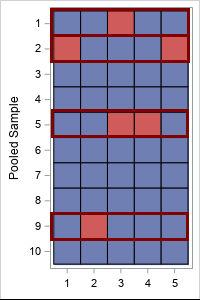Suppose you have 50 samples that you want to test. If you test them individually, you need 50 tests. But suppose you combine five samples at a time into 10 pooled samples. In the first round of testing, you use 10 tests on the pooled samples. Suppose four of the tests are positive. In the second round of testing, you test the 4*5 = 20 samples that were in the four pooled samples. The total number of tests used is 30: 10 for the first round and 20 for the second round. You have tested the same number of samples while running 60% of the tests.

This is illustrated by the table at the right. The individual samples are the cells of the table. Blue is a negative sample; red is a positive sample. The rows represent the 10 pooled samples. The four highlighted rows (rows 1, 2, 5, and 9) indicate the pooled samples that test positive in the first round. The 20 samples in those rows must be tested individually in the second round.

Pool testing is most efficient when the probability of an infected sample is small. That enables you to pool many individuals at one time.

### How many tests are saved by using pool testing?

In the previous example, 50 individuals were tested by using 30 tests. That means that pool testing (with five samples in each pool) used only 60% of the tests as individual testing. The proportion of tests that you need is related to the probability of an infected sample (p) and how many tests (k) you combine to make a pooled sample.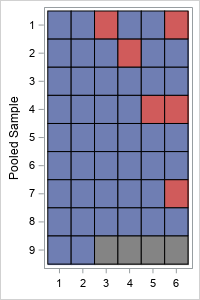The lab that performs the tests cannot control the probability of an infected sample (p), but they can control the number of samples (k) in each pool. What happens if you change k? Let's revisit the previous example, but use six samples in each pool. The pooled-sample design for k=6 is shown to the right. There are nine pooled samples (rows) so nine tests are required for the first round. (Because 6 does not evenly divide 50, the last pooled sample only contains two samples.) Four pooled samples (rows 1, 2, 4, and 7) test positive, which triggers 4*k = 4*6 = 24 additional tests in the second round. So the total number of tests when k=6 is 9 + 24 = 33 tests.

Although pooling k=6 samples resulted in more total tests than for k=5 for these data, there is randomness in this result. The order of the samples and the number of positive samples are random and unknowable. Nevertheless, you can use probability theory to predict the expected number of tests that you will need in a random sample when you use k samples in each pool.

### How many samples should you combine to make a pooled sample?

If you know that the probability (p) that a sample is infected, you can compute the optimal number of samples (k) to combine into a pooled sample. Here "optimal" means "resulting in the fewest tests, on average."

Here comes the math. Suppose you want to test a large number, N, of individual samples. If each pooled sample contains k individual samples, then:

• There are about N/k pooled samples, so you need that many tests for the first round of testing.
• For each pooled sample, the probability that the sample does NOT test positive is the probability of having zero positive samples in a random set of k indpendent samples. This probability is given by the binomial distribution: Binom(0, p, k) = (1 – p)k. Consequently, the probability that a pooled sample DOES test positive is
p2 = 1 – (1 – p)k.
• From the preceding calculation, the expected number of positive pooled samples is p2N/k.
• Each positive test from the first round triggers k additional tests in the second round, so the expected number of tests in the second round is p2N.
• Consequently, the expected number of TOTAL tests is NTot = N(1/k + p2).
• If you don't use pooling, you have to do N tests, so pool testing reduces the total number of tests by the expected fraction 1/k + p2 or
f(k; p) = 1/k + 1 + (1 – p)k.

In summary, if p is the probability that a sample is infected, and you combine k samples into each pool, expected reduction in tests is the proportion 1/k + 1 + (1 – p)k.

The lab can control k, the number of samples that are combined into each pooled sample. So what value of k is expected to cost the fewest number of tests? The following graph shows a graph of the expected proportion as a function of k for several value of the proportion p.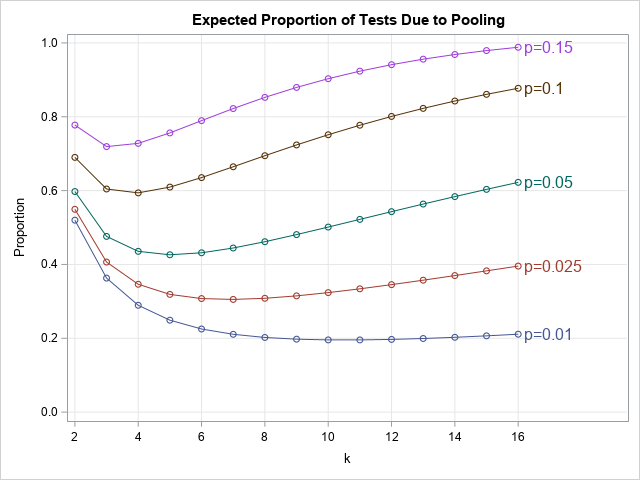The graph shows the following:

• When p=0.15 (15% of tests are expected to be positive), the optimal value is k=3, and you can expect to need 72% of the tests compared to testing each individual sample.
• When p=0.1 (10% of tests are expected to be positive), the optimal value is k=4, and you need about 59% of the tests compared to not pooling.
• When p=0.05 (5%), the optimal value is k=5, and you need about 43% of the tests compared to not pooling.
• When p=0.025 (2.5%), the optimal value is k=7, and you need about 31% of the tests.
• When p=0.01 (1%), the optimal value is k=11, and you need about 20% of the tests.

In this section, I focused on testing the same number of people with fewer tests. But you can also test more people with the same number of tests. For example, when p=0.01, you can either test the same number of people using 20% of the tests, or you can test five times more people (because 1/0.2 = 5) with the same number of tests.

### Can pool testing reduce tests 'tenfold'?

In the USA Today article, epidemiologists are quoted as saying that "pool testing has the potential to increase the number of people tested tenfold, 'if not 100-fold.'” Notice that none of the curves in this article indicate an increase that large. The largest increase is for p=0.01 and k=11, which is a fivefold increase.

Pool testing is most effective when the probability of a positive test is very small. A small probability occurs when you test a mostly healthy population as a way of monitoring health and preventing future outbreaks. If the test is very sensitive, you could theoretically pool dozens or hundreds of samples together, which could lead to a dramatic increase in the number of people that you can test. I do not know the sensitivity of the COVID-19 tests in the US, but there is a practical limit on how many samples can be pooled.

Currently, many tests in the US are given to people who are exhibiting symptoms or believe they might have been exposed. For example, in North Carolina, the current percentage of positive tests is 6%. That is also the approximate national average. Some of the hardest-hit US states (Arizona, Florida, Texas,...) are reporting positive test rates closer to 10% or 15% or more, as reported by the Johns Hopkins data on "Daily State-by-State Testing Trends." Unfortunately, the hardest-hit states (where there is a high probability of a positive test) benefit the least from pooling.

In conclusion, this article shows that you can use pool testing to reduce the number of tests required to test a large number of people. If applied to the current testing in the US, it could reduce the number of tests 30% to 80%. (Equivalently, you could test 1.25 to 3.3 times as many people.) If applied to a mostly healthy population, it could reduce the number of tests even more. (Equivalently, test many more people.) This article is about the mathematics of pool testing and does not consider economic factors or practical considerations.

The post Pool testing: The math behind combining medical tests appeared first on The DO Loop.The first time I saw a formula for the pooled variance, I was quite confused. It looked like Frankenstein's monster, assembled from bits and pieces of other quantities and brought to life by a madman. However, the pooled variance does not have to be a confusing monstrosity. The verb "to pool" means to combine resources from several sources, as in, "if the siblings pool their money, they can buy a nice gift for their mother." Thus a "pooled variance" combines information from several subgroups of the data in order to obtain a better estimate. This article shows how to understand the pooled variance, which is an average of the sample variances for subgroups of the data.

The following graph visualizes the pooled variance and the variances within groups. In the graph, there are three groups. The sample variance within each group is plotted as a blue marker. The pooled variance is indicated by a horizontal line. The pooled variance appears to be an average of the three sample variances. The exact formulas and the data for this graph are explained in subsequent sections.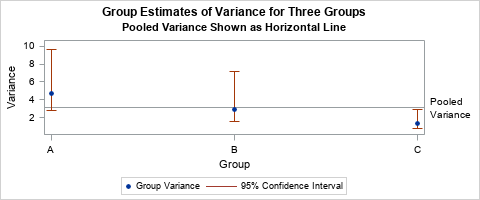### The pooled variance is an average of group variances

Most students first encounter the pooled variance in a statistics course when learning about two-sample t tests. In a two-sample t test, you have data in two groups and you want to test whether the means of the two groups are different. In order to run a two-sample t test, you need to decide whether you think the variances of the two groups are equal. If you think the group variances are equal, you compute the pooled variance, which estimates the common variance. You use the pooled variance estimate to compute the t statistic.

The pooled variance combines (or "pools") the variance estimates within the individual groups. The pooled variance is a better estimate of the (unknown) common group variance than either of the individual group variances. If each group has the same number of observations, then the pooled variance is a simple average. If the group sizes are different, then the pooled variance is a weighted average, where larger groups receive more weight than smaller groups.

For the two-sample case, let $s_1^2$ and $s_2^2$ be the sample variances within the groups A and B, respectively. Let $n_1$ and $n_2$, respectively, be the number of observations in the groups. If you assume that the data in each group have the same variance, how can you estimate that common variance? One way is to "pool" the variances of each group and compute a weighted average of the variances. For two groups, the pooled variance is
$s_p^2 = \frac{(n_1-1)s_1^2 + (n_2-1)s_2^2}{n_1 + n_2- 2}$
The numerator is the weighted sum of the group variances. Dividing by the sum of the weights means that the pooled variance is the weighted average of the two quantities.

Notice that if $n_1 = n_2$, then the formula simplifies. When the group sizes are equal, the pooled variance reduces to $s_p^2 = (s_1^2 + s_2^2)/2$, which is the average of the two variances.

The idea behind the pooled variance generalizes to multiple groups. Suppose you collect data for $k$ groups and $s_i^2$ is the sample variance within the $i$th group, which has $n_i$ observations. If you believe that the groups have a common variance, how might you estimate it? One way is to compute the pooled variance as a weighted average of the group variances:
$s_p^2 = \Sigma_{i=1}^k (n_i-1)s_i^2 / \Sigma_{i=1}^k (n_i - 1)$
Again, if all groups have the same number of observations, then the formula simplifies to $\Sigma_{i=1}^k s_i^2 / k$, which is the average of the $k$ variances.

### Visualize the pooled variance

Formulas are necessary for computation, but I like to think in pictures. You can draw a graph that shows how the pooled variance relates to the variances of the groups.

Let's use an example that has three groups: A, B, and C. The following data (adapted from an example in the SAS documentation) are the ozone measurements (Y, in parts per billion) at three different collection sites (A, B, and C):

Site n Ozone (ppb) ==== === ============================================= A 22 4 6 3 4 7 8 2 3 4 1 3 8 9 5 6 4 6 3 4 7 3 7 B 15 5 3 6 2 1 2 4 3 2 4 6 4 6 3 6 C 18 8 9 7 8 6 7 6 7 9 8 9 8 7 8 5 8 9 7

The following graph shows a box plot of the ozone levels at each site. The height of each box gives a rough estimate of the variance in each group. The mean and variance for sites A and B appear to be similar to each other. However, the mean at site C appears to be larger and the variance seems smaller, compared to the other sites.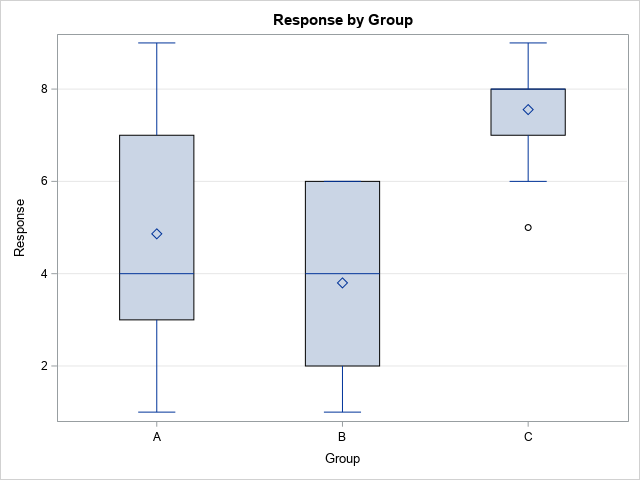You can use your favorite statistical software to estimate the variance of the data for each site. The following table is produced in SAS by using the UNIVARIATE procedure and the CIBASIC option: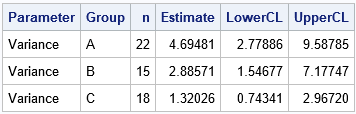The table shows an estimate for the variance of the data within each group. Although the smallest sample variance (Group C: 1.32) seems much smaller than the largest sample variance (Group A: 4.69), notice that the 95% confidence intervals overlap. A parameter value such as 2.8 or 2.9 would simultaneously be in all three confidence intervals. If we assume that the variance of the groups are equal, the pooled variance formula provides a way to estimate the common variance. Applying the formula, the estimate for the pooled variance for these data is
$s_p^2 = (21*4.69 + 14*2.89 + 17*1.32) / (21+14+17) = 3.10$

The graph at the top of this article visualizes the information in the table and uses a reference line to indicate the pooled variance. The blue markers indicate the sample variances of each group. The confidence intervals for the population variances are shown by the vertical lines. The pooled variance is indicated by the horizontal reference line. It is the weighted average of the sample variances. If you think that all groups have the same variance, the pooled variance estimates that common variance.

### Summary

In two-sample t tests and ANOVA tests, you often assume that the variance is constant across different groups in the data. The pooled variance is an estimate of the common variance. It is a weighted average of the sample variances for each group, where the larger groups are weighted more heavily than smaller groups.

You can download a SAS program that computes the pooled variance and creates the graphs in this article. Although the pooled variance provides an estimate for a common variance among groups, it is not always clear when the assumption of a common variance is valid. The visualization in this article can help, or you can perform a formal "homogeneity of variance" test as part of an ANOVA analysis.

The post What is a pooled variance? appeared first on The DO Loop.Every day we face risks. If we drive to work, we risk a fatal auto accident. If we eat red meat and fatty foods, we risk a heart attack. If we go out in public during a pandemic, we risk contracting a disease. A logical response to risk is to mitigate the risk by making healthy choices. Don't text while driving. Modify your diet. Stay at home as much as possible during a pandemic.

Unfortunately, sometimes individuals do not make wise choices. During the early stages of the coronavirus outbreak, there were reports of people ignoring social-distancing guidelines and aggregating on beaches and in bars. Rick McAnulty, a psychologist and professor of psychology at UNC-Charlotte, states that noncompliance with health-related issues is widespread and is not limited to the coronavirus pandemic. In general, he says, "People estimate the probability of risk—and decide the odds outweigh probable danger."

But how do people "estimate the probability" of a risk? Unless you are an expert in traffic accidents, heart disease, or viruses, a realistic estimate is too complicated for most of us. However, there is a simple probability model that demonstrates how the probability of a disastrous result increases due to repeated exposure to risk. In simple terms, if doing some action might lead to a bad result, you can reduce your overall risk by

• doing that action less often
• reducing the probability that the action leads to the bad result

Yes, this is common sense, but you can also demonstrate these facts mathematically by using a probability model, as shown in subsequent sections. I will use the spread of coronavirus as a motivating example, but be aware that I am a statistician, not an expert in infectious diseases.

### A statistical model for risk

During the coronavirus pandemic, public-health experts advocate modifying our behavior to reduce the risk of catching and spreading the disease. The public-health directives can be classified into two broad categories:

• Reduce exposure: Some directives limit the number of interactions between susceptible people and infected people. These include closing places where people gather, limiting the size of groups, social distancing, and staying at home except for urgent needs.
• Reduce transmission: Other directives reduce the probability that an interaction between a susceptible person and an infected person will transmit the virus. These include washing hands, disinfecting surfaces, and wearing face masks. (Personal protective equipment, worn by health care workers, are also in this category.)

It is fortunate that the same social distancing that protects us from others also protects others from us!

You can use a simple probability model, called the "geometric distribution," to demonstrate why these directives are effective. The geometric distribution uses two terms that might be unfamiliar. A trial is an experiment that has two outcomes. One of the outcomes is labeled the event. The geometric distribution assumes that the trials are independent and that the event has the same probability of happening for each trial. I wrote a statistical article about the geometric distribution.

For example, you could define a "trial" to mean "go to the grocery store" and the "event" to mean "become infected with the coronavirus." There is a probability that the event occurs for each trial, and the model assumes that the probability is the same for each trial. Different activities might have different probabilities. Going to work might be riskier than going to the store, which might be riskier than a walk in the neighborhood.

If you know the probability that an event can happen for one trial, the cumulative geometric distribution gives the probability that it will happen on or before the nth trial, for n = 1, 2, 3, .... For example, if you specify the probability that someone becomes infected during an activity, the cumulative distribution gives the probability that the person will become infected if he repeats the activity one time, two times, and so forth.

### The cumulative distribution of the geometric model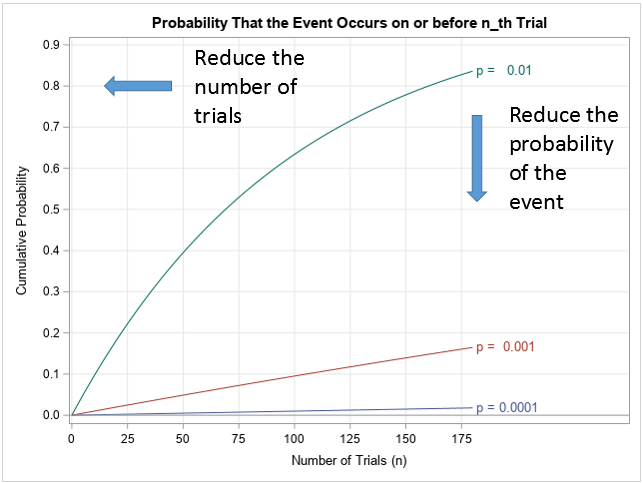The graph to the right shows the cumulative probability as a function of n (the number of trials) for three hypothetical activities. (Click to enlarge.) For one activity (green curve, top), the probability that the event occurs for each trial is p=0.01 or 1%. The values along the curve show the probability that the event happens on or before the nth trial for n = 1, 2, 3, .... For a different activity (red curve, middle), the probability for each trial is only p=0.001 or 0.1%. For a third activity (blue curve, bottom), the probability is extremely small: p=0.0001 or 0.01%. These probability values are for illustration purposes only; they do not represent any specific activity that is related to contracting coronavirus.

There are three distinguishing features of the curves:

1. For a specified event probability, the cumulative probability increases with the number of trials. Thus, the geometric distribution shows that you can reduce the cumulative probability of an event by reducing the number of trials.
2. For a specified number of trials, the cumulative probability is lower when the underlying event probability is lower. Thus, if you can reduce the underlying probability of the event, you can perform the same number of trials with less risk.
3. For very small probabilities, you can quantify how much the cumulative probability increases with each additional trial. Notice that the bottom curves look almost linear. As shown in my previous article, the slope of each curve is close to p. Thus, in the geometric distribution model, each additional trial increases the cumulative probability by approximately p. The chance that the event occurs on the first, second, and third trials is approximately p, 2p, and 3p, respectively.

As mentioned previously, public-health officials urge each individual to limit the number of person-to-person interactions, if possible. Unfortunately, some people (such as health care workers and grocery clerks) cannot control the number of people with whom they interact. Thus, the directives also instruct those workers (and we who interact with them!) to use other methods to reduce the probability of the underlying event (infection). Taken together, the directives try to move each member of the population "left and down" towards the lower-left corner of the graph, where the probability of infection is the lowest.

### With random events, sometimes you are unlucky

If you hear that there is only a 1% chance that some random event will occur, you might be falsely lured into thinking you can take that risk about 100 times before you need to worry about it. THAT IS NOT TRUE. No matter how small the probability is, the event can happen on the first trial, or the sixth, or the tenth. You can't predict when an event will happen.

In a previous article, I showed how to simulate a random sample from the geometric distribution. One such sample is shown below for p=0.01. The graph represents 20 subjects who each repeated a trial until the event occurred. The graph shows an ordered list of the number of trials for the 20 subjects: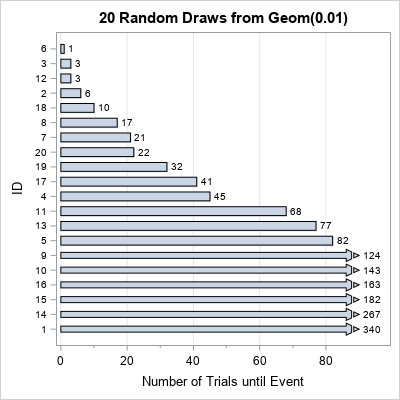Of the 20 subjects, five experienced the event in 10 or fewer trials. One experienced the event on the first trial. These subjects were unlucky. There were also lucky subjects. For six subjects, the event did not occur before the 100th trial. On average, the expected number of trials until the event is 100, but there is large variation in these numbers. A small probability does not mean that the event will not happen.

### Do we know the probability of catching coronavirus?

I will not attempt to assign a probability to "the event" of catching the coronavirus while engaging in everyday activities. Other mathematicians have made estimates earlier in the pandemic, and you can read about their efforts in the links below:

### Conclusions

The geometric distribution isn't complex enough to be realistic, and we don't have good estimates for the probability of catching the coronavirus, so what good is this simple model? Well, as the statistician George Box famously said, "All models are wrong, but some are useful." Even simple models can provide a qualitative description of the consequences of certain actions. You can use the cumulative geometric distribution to understand how actions under your control (social distancing, washing hands, ....) affect the probability of spreading coronavirus to yourself and to others. Specifically, the model supports the basic advice from health care professionals:

• Reduce your exposure. This moves you "to the left" in the graph of the geometric distribution model. Health care directives that limit interactions include staying at home when possible and practicing social distancing when you venture out.
• Reduce the probability of transmission. This moves you "down" in the graph of the geometric distribution model. Directives include washing hands, disinfecting surfaces, and wearing masks.

In addition, for very small probabilities, the geometric model shows that the cumulative probability of an event is approximately proportional to the number of trials. This quantifies how much each additional exposure increases the risk of transmission. Finally, the geometric distribution teaches a grim lesson: a small probability is still a nonzero. In a simulation of a random sample from the geometric distribution, there are usually individuals who experienced the event after only a few trials.

So help yourself and others by following the directives of health care professionals. The strategies they advocate are supported by science, common sense, and the mathematics of probability theory.

The post On reducing the spread of coronavirus appeared first on The DO Loop.During an outbreak of a disease, such as the coronavirus (COVID-19) pandemic, the media shows daily graphs that convey the spread of the disease. The following two graphs appear frequently:

• New cases for each day (or week). This information is usually shown as a histogram or needle plot. The graph is sometimes called a frequency graph.
• The total number of cases plotted against time. Usually, this graph is a line graph. The graph is sometimes called a cumulative frequency graph.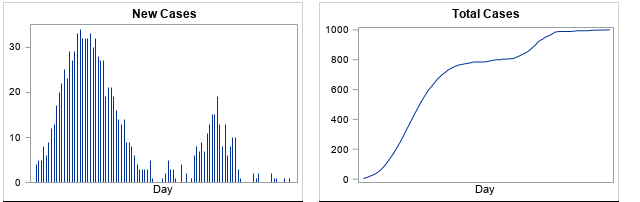An example of each graph is shown above. The two graphs are related and actually contain the same information. However, the cumulative frequency graph is less familiar and is harder to interpret. This article discusses how to read a cumulative frequency graph. The shape of the cumulative curve indicates whether the daily number of cases is increasing, decreasing, or staying the same.

For this article, I created five examples that show the spread of a hypothetical disease. The numbers used in this article do not reflect any specific disease or outbreak.

### How to read a cumulative frequency graph

When the underlying quantity is nonnegative (as for new cases of a disease), the cumulative curve never decreases. It either increases (when new cases are reported) or it stays the same (if no new cases are reported).

When the underlying quantity (new cases) is a count, the cumulative curve is technically a step function, but it is usually shown as a continuous curve by connecting each day's cumulative total. A cumulative curve for many days (more than 40) often looks smooth, so you can describe its shape by using the following descriptive terms: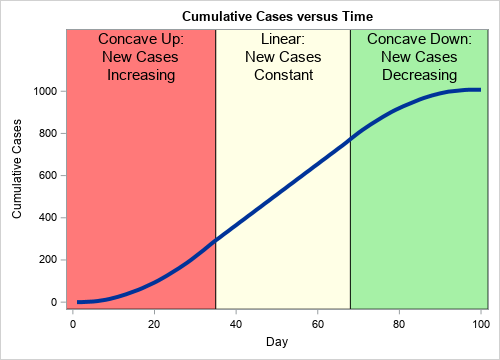• When the number of new cases is increasing, the cumulative curve is "concave up." In general, a concave-up curve is U-shaped, like this: ∪. Because a cumulative frequency curve is nondecreasing, it looks like the right side of the ∪ symbol.
• When the number of new cases is staying the same, the cumulative curve is linear. The slope of the curve indicates the number of new cases.
• When the number of new cases is decreasing, the cumulative curve is "concave down." In general, a concave-up curve looks like an upside-down U, like this: ∩. Because a cumulative frequency curve is nondecreasing, a concave-down curve looks like the left side of the ∩ symbol.

A typical cumulative curve is somewhat S-shaped, as shown to the right. The initial portion of the curve (the red region) is concave up, which indicates that the number of new cases is increasing. The cumulative curve is nearly linear between Days 35 and 68 (the yellow region), which indicates that the number of new cases each day is approximately constant. After Day 68, the cumulative curve is concave down, which indicates that the number of daily cases is decreasing. Each interval can be short, long, or nonexistent.

The cumulative curve looks flat near Day 100. When the cumulative curve is exactly horizontal (zero slope), it indicates that there are no new cases.

Sometimes you might see a related graph that displayed the logarithm of the cumulative cases. Near the end of this article, I briefly discuss how to interpret a log-scale graph.

### Examples of frequency graphs

It is useful to look at the shape of the cumulative frequency curve for five different hypothetical scenarios. This section shows the cases-per-day frequency graphs; the cumulative frequency curves are shown in subsequent sections.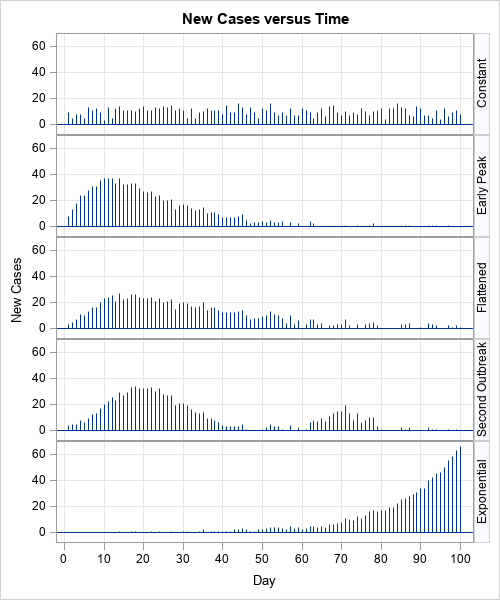In each scenario, a population experiences a total of 1,000 cases of a disease over a 100-day time period. For the sake of discussion, suppose that the health care system can treat up to 20 new cases per day. The graphs to the right indicate that some scenarios will overwhelm the health care system whereas others will not. The five scenarios are:

• Constant rate of new cases: In the top graph, the community experiences about 10 new cases per day for each of the 100 days. Because the number of cases per day is small, the health care system can treat all the infected cases.
• Early peak: In the second graph, the number of new cases quickly rises for 10 days before gradually declining over the next 50 days. Because the more than 20 new cases develop on Days 5–25, the health care system is overwhelmed on those days.
• Delayed peak: In the third graph, the number of new cases gradually rises, levels out, and gradually declines. There are only a few days in which the number of new cases exceeds the capacity of the health care system. Epidemiologists call this scenario "flattening the curve" of the previous scenario. By practicing good hygiene and avoiding social interactions, a community can delay the spread of a disease.
• Secondary outbreak: In the fourth graph, the first outbreak is essentially resolved when a second outbreak appears. This can happen, for example, if a new infected person enters a community after the first outbreak ends. To prevent this scenario, public health officials might impose travel bans on certain communities.
• Exponential growth: In the fifth graph, the number of new cases increases exponentially. The health care system is eventually overwhelmed, and the graph does not indicate when the spread of the disease might end.

The graphs in this section are frequency graphs. The next sections show and interpret a cumulative frequency graph for each scenario.

### Constant rate of new cases

In the first scenario, new cases appear at a constant rate. Consequently, the cumulative frequency chart looks like a straight line. The slope of the line is the rate at which new cases appear. For example, in this scenario, the number of new cases each day is approximately 10. Consequently, the cumulative curve has an average slope ("rise over run") that is close to 10.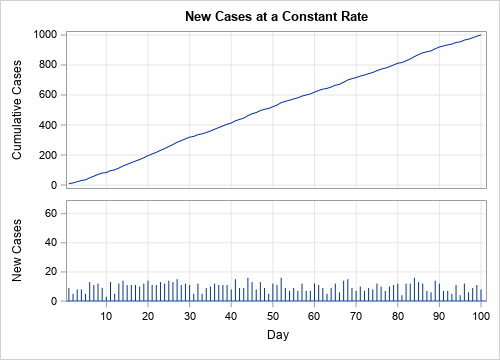### Early peak

In the second scenario, new cases appear very quickly at first, then gradually decline. Consequently, the first portion of the cumulative curve is concave up and the second portion is concave down. In this scenario, the number of new cases dwindles to zero, as indicated by the nearly horizontal cumulative curve.

At any moment in time, you can use the slope of the cumulative curve to estimate the number of new cases that are occurring at that moment. Days when the slope of the cumulative curve is large (such as Day 10), correspond to days on which many new cases are reported. Where the cumulative curve is horizontal (zero slope, such as after Day 60), there are very few new cases.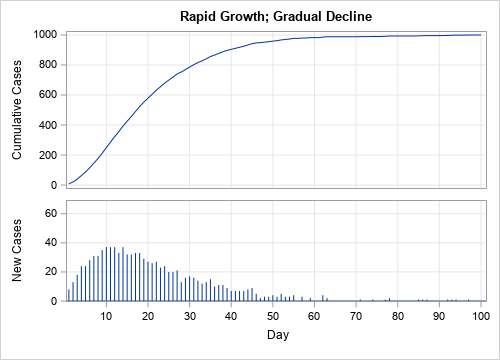### Delayed peak

In the third scenario, new cases appear gradually, level out, and then decline. This is reflected in the cumulative curve. Initially, the cumulative curve is concave up. It then straightens out and appears linear for 10–15 days. Finally, it turns concave down, which indicates that the number of new cases is trending down. Near the end of the 100-day period, the cumulative curve is nearly horizontal because very few new cases are being reported.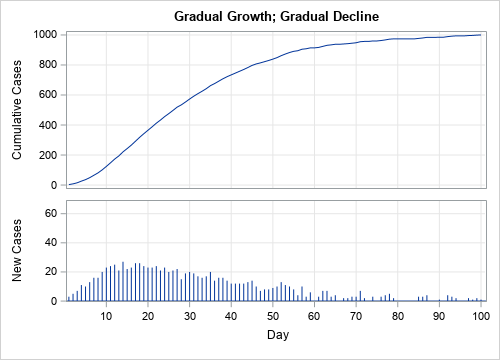### Secondary outbreak

In the fourth scenario, there are two outbreaks. During the first outbreak, the cumulative curve is concave up or down as the new cases increase or decrease, respectively. The cumulative curve is nearly horizontal near Day 50, but then goes through a smaller concave up/down cycle as the second outbreak appears. Near the end of the 100-day period, the cumulative curve is once again nearly horizontal as the second wave ends.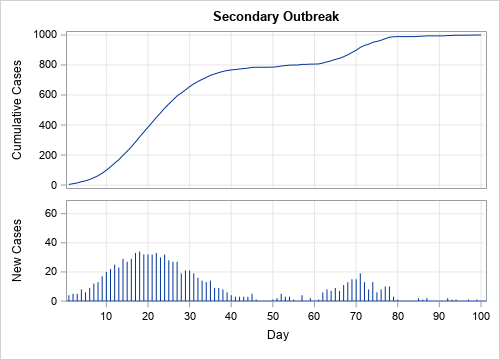### Exponential growth

The fifth scenario demonstrates exponential growth. Initially, the number of new cases increases very gradually, as indicated by the small slope of the cumulative frequency curve. However, between Days 60–70, the number of new cases begins to increase dramatically. The lower and upper curves are both increasing at an exponential rate, but the scale of the vertical axis for the cumulative curve (upper graph) is much greater than for the graph of new cases (lower graph). This type of growth is more likely in a population that does not use quarantines and "social distancing" to reduce the spread of new cases.

This last example demonstrates why it is important to label the vertical axis. At first glance, the upper and lower graphs look very similar. Both exhibit exponential growth. One way to tell them apart is to remember that a cumulative frequency graph never decreases. In contrast, if you look closely at the lower graph, you can see that some bars (Days 71 and 91) are shorter than the previous day's bar.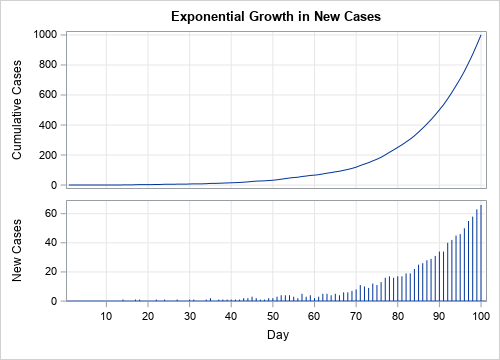### Be aware of log-scale axes

The previous analysis assumes that the vertical axis plot the cumulative counts on a linear scale. Scientific articles might display the logarithm of the total counts. The graph is on a log scale if the vertical axis says "log scale" or if the tick values are powers of 10 such as 10, 100, 1000, and so forth. If the graph uses a log scale:

• A straight line indicates that new cases are increasing at an exponential rate. The slope of the line indicates how quickly cases will double, with steep lines indicating a short doubling time.
• A concave-down curve indicates that new cases are increasing at rate that is less than exponential. Log-scale graphs make it difficult to distinguish between a slowly increasing rate and a decreasing rate.

### Summary

In summary, this article shows how to interpret a cumulative frequency graph. A cumulative frequency graph is provided for five scenarios that describe the spread of a hypothetical disease. In each scenario, the shape of the cumulative frequency graph indicates how the disease is spreading:

• When the cumulative curve is concave up, the number of new cases is increasing.
• When the cumulative curve is linear, the number of new cases is not changing.
• When the cumulative curve is concave down, the number of new cases is decreasing.
• When the cumulative curve is horizontal, there are no new cases being reported.

Although the application in this article is the spread of a fictitious disease, the ideas apply widely. Anytime you see a graph of a cumulative quantity (sales, units produced, number of traffic accidents,...), you can the ideas in this article to interpret the cumulative frequency graph and use its shape to infer the trends in the underlying quantity. Statisticians use these ideas to relate a cumulative distribution function (CDF) for a continuous random variable to its probability density function (PDF).

The post How to read a cumulative frequency graph appeared first on The DO Loop.During an outbreak of a disease, such as the coronavirus (COVID-19) pandemic, the media shows daily graphs that convey the spread of the disease. The following two graphs appear frequently:

• New cases for each day (or week). This information is usually shown as a histogram or needle plot. The graph is sometimes called a frequency graph.
• The total number of cases plotted against time. Usually, this graph is a line graph. The graph is sometimes called a cumulative frequency graph.An example of each graph is shown above. The two graphs are related and actually contain the same information. However, the cumulative frequency graph is less familiar and is harder to interpret. This article discusses how to read a cumulative frequency graph. The shape of the cumulative curve indicates whether the daily number of cases is increasing, decreasing, or staying the same.

For this article, I created five examples that show the spread of a hypothetical disease. The numbers used in this article do not reflect any specific disease or outbreak.

### How to read a cumulative frequency graph

When the underlying quantity is nonnegative (as for new cases of a disease), the cumulative curve never decreases. It either increases (when new cases are reported) or it stays the same (if no new cases are reported).

When the underlying quantity (new cases) is a count, the cumulative curve is technically a step function, but it is usually shown as a continuous curve by connecting each day's cumulative total. A cumulative curve for many days (more than 40) often looks smooth, so you can describe its shape by using the following descriptive terms:• When the number of new cases is increasing, the cumulative curve is "concave up." In general, a concave-up curve is U-shaped, like this: ∪. Because a cumulative frequency curve is nondecreasing, it looks like the right side of the ∪ symbol.
• When the number of new cases is staying the same, the cumulative curve is linear. The slope of the curve indicates the number of new cases.
• When the number of new cases is decreasing, the cumulative curve is "concave down." In general, a concave-up curve looks like an upside-down U, like this: ∩. Because a cumulative frequency curve is nondecreasing, a concave-down curve looks like the left side of the ∩ symbol.

A typical cumulative curve is somewhat S-shaped, as shown to the right. The initial portion of the curve (the red region) is concave up, which indicates that the number of new cases is increasing. The cumulative curve is nearly linear between Days 35 and 68 (the yellow region), which indicates that the number of new cases each day is approximately constant. After Day 68, the cumulative curve is concave down, which indicates that the number of daily cases is decreasing. Each interval can be short, long, or nonexistent.

The cumulative curve looks flat near Day 100. When the cumulative curve is exactly horizontal (zero slope), it indicates that there are no new cases.

Sometimes you might see a related graph that displayed the logarithm of the cumulative cases. Near the end of this article, I briefly discuss how to interpret a log-scale graph.

### Examples of frequency graphs

It is useful to look at the shape of the cumulative frequency curve for five different hypothetical scenarios. This section shows the cases-per-day frequency graphs; the cumulative frequency curves are shown in subsequent sections.In each scenario, a population experiences a total of 1,000 cases of a disease over a 100-day time period. For the sake of discussion, suppose that the health care system can treat up to 20 new cases per day. The graphs to the right indicate that some scenarios will overwhelm the health care system whereas others will not. The five scenarios are:

• Constant rate of new cases: In the top graph, the community experiences about 10 new cases per day for each of the 100 days. Because the number of cases per day is small, the health care system can treat all the infected cases.
• Early peak: In the second graph, the number of new cases quickly rises for 10 days before gradually declining over the next 50 days. Because the more than 20 new cases develop on Days 5–25, the health care system is overwhelmed on those days.
• Delayed peak: In the third graph, the number of new cases gradually rises, levels out, and gradually declines. There are only a few days in which the number of new cases exceeds the capacity of the health care system. Epidemiologists call this scenario "flattening the curve" of the previous scenario. By practicing good hygiene and avoiding social interactions, a community can delay the spread of a disease.
• Secondary outbreak: In the fourth graph, the first outbreak is essentially resolved when a second outbreak appears. This can happen, for example, if a new infected person enters a community after the first outbreak ends. To prevent this scenario, public health officials might impose travel bans on certain communities.
• Exponential growth: In the fifth graph, the number of new cases increases exponentially. The health care system is eventually overwhelmed, and the graph does not indicate when the spread of the disease might end.

The graphs in this section are frequency graphs. The next sections show and interpret a cumulative frequency graph for each scenario.

### Constant rate of new cases

In the first scenario, new cases appear at a constant rate. Consequently, the cumulative frequency chart looks like a straight line. The slope of the line is the rate at which new cases appear. For example, in this scenario, the number of new cases each day is approximately 10. Consequently, the cumulative curve has an average slope ("rise over run") that is close to 10.### Early peak

In the second scenario, new cases appear very quickly at first, then gradually decline. Consequently, the first portion of the cumulative curve is concave up and the second portion is concave down. In this scenario, the number of new cases dwindles to zero, as indicated by the nearly horizontal cumulative curve.

At any moment in time, you can use the slope of the cumulative curve to estimate the number of new cases that are occurring at that moment. Days when the slope of the cumulative curve is large (such as Day 10), correspond to days on which many new cases are reported. Where the cumulative curve is horizontal (zero slope, such as after Day 60), there are very few new cases.### Delayed peak

In the third scenario, new cases appear gradually, level out, and then decline. This is reflected in the cumulative curve. Initially, the cumulative curve is concave up. It then straightens out and appears linear for 10–15 days. Finally, it turns concave down, which indicates that the number of new cases is trending down. Near the end of the 100-day period, the cumulative curve is nearly horizontal because very few new cases are being reported.### Secondary outbreak

In the fourth scenario, there are two outbreaks. During the first outbreak, the cumulative curve is concave up or down as the new cases increase or decrease, respectively. The cumulative curve is nearly horizontal near Day 50, but then goes through a smaller concave up/down cycle as the second outbreak appears. Near the end of the 100-day period, the cumulative curve is once again nearly horizontal as the second wave ends.### Exponential growth

The fifth scenario demonstrates exponential growth. Initially, the number of new cases increases very gradually, as indicated by the small slope of the cumulative frequency curve. However, between Days 60–70, the number of new cases begins to increase dramatically. The lower and upper curves are both increasing at an exponential rate, but the scale of the vertical axis for the cumulative curve (upper graph) is much greater than for the graph of new cases (lower graph). This type of growth is more likely in a population that does not use quarantines and "social distancing" to reduce the spread of new cases.

This last example demonstrates why it is important to label the vertical axis. At first glance, the upper and lower graphs look very similar. Both exhibit exponential growth. One way to tell them apart is to remember that a cumulative frequency graph never decreases. In contrast, if you look closely at the lower graph, you can see that some bars (Days 71 and 91) are shorter than the previous day's bar.### Be aware of log-scale axes

The previous analysis assumes that the vertical axis plot the cumulative counts on a linear scale. Scientific articles might display the logarithm of the total counts. The graph is on a log scale if the vertical axis says "log scale" or if the tick values are powers of 10 such as 10, 100, 1000, and so forth. If the graph uses a log scale:

• A straight line indicates that new cases are increasing at an exponential rate. The slope of the line indicates how quickly cases will double, with steep lines indicating a short doubling time.
• A concave-down curve indicates that new cases are increasing at rate that is less than exponential. Log-scale graphs make it difficult to distinguish between a slowly increasing rate and a decreasing rate.

### Summary

In summary, this article shows how to interpret a cumulative frequency graph. A cumulative frequency graph is provided for five scenarios that describe the spread of a hypothetical disease. In each scenario, the shape of the cumulative frequency graph indicates how the disease is spreading:

• When the cumulative curve is concave up, the number of new cases is increasing.
• When the cumulative curve is linear, the number of new cases is not changing.
• When the cumulative curve is concave down, the number of new cases is decreasing.
• When the cumulative curve is horizontal, there are no new cases being reported.

Although the application in this article is the spread of a fictitious disease, the ideas apply widely. Anytime you see a graph of a cumulative quantity (sales, units produced, number of traffic accidents,...), you can the ideas in this article to interpret the cumulative frequency graph and use its shape to infer the trends in the underlying quantity. Statisticians use these ideas to relate a cumulative distribution function (CDF) for a continuous random variable to its probability density function (PDF).

The post How to read a cumulative frequency graph appeared first on The DO Loop.Books about statistics and machine learning often discuss the tradeoff between bias and variance for an estimator. These discussions are often motivated by a sophisticated predictive model such as a regression or a decision tree. But the basic idea can be seen in much simpler situations. This article presents a simple situation that is discussed in a short paper by Dan Jeske (1993). Namely, if a random process produces integers with a known set of probabilities, what method should you use to predict future values of the process?

I will start by briefly summarizing Jeske's result, which uses probability theory to derive the best biased and unbiased estimators. I then present a SAS program that simulates the problem and compares two estimators, one biased and one unbiased.

### A random process that produces integers

Suppose a gambler asks you to predict the next roll of a six-sided die. He will reward you based on how close your guess is to the actual value he rolls. No matter what number you pick, you only have a 1/6 chance of being correct. But if the strategy is to be close to the value rolled, you can compute the expected value of the six faces, which is 3.5. Assuming that the gambler doesn't let you guess 3.5 (which is not a valid outcome), one good strategy is to round the expected value to the nearest integer. For dice, that means you would guess ROUND(3.5) = 4. Another good strategy is to randomly guess either 3 or 4 with equal probability.

Jeske's paper generalizes this problem. Suppose a random process produces the integers 1, 2, ..., N, with probabilities p1, p2, ..., pN, where the sum of the probabilities is 1. (This random distribution is sometimes called the "table distribution.") If your goal is to minimize the mean squared error (MSE) between your guess and a series of future random values, Jeske shows that the optimal solution is to guess the value that is closest to the expected value of the random variable. I call this method the ROUNDING estimator. In general, this method is biased, but it has the smallest expected MSE. Recall that the MSE is a measure of the quality of an estimator (smaller is better).

An alternative method is to randomly guess either of the two integers that are closest to the expected value, giving extra weight to the integer that is closer to the expected value. I call this method the RANDOM estimator. The random estimator is unbiased, but it has a higher MSE.

### An example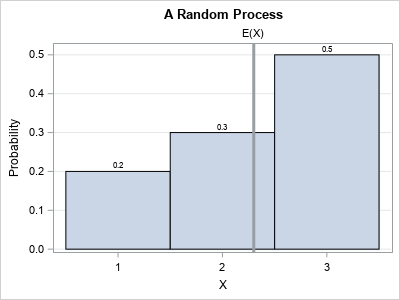The following example is from Jeske's paper. A discrete process generates a random variable, X, which can take the values 1, 2, and 3 according to the following probabilities:

• P(X=1) = 0.2, which means that the value 1 appears with probability 0.2.
• P(X=2) = 0.3, which means that the value 2 appears with probability 0.3.
• P(X=3) = 0.5, which means that the value 3 appears with probability 0.5.

A graph of the probabilities is shown to the right. The expected value of this random variable is E(X) = 1(0.2) + 2(0.3) + 3(0.5) = 2.3. However, your guess must be one of the feasible values of X, so you can't guess 2.3. The best prediction (in the MSE sense) is to round the expected value. Since ROUND(2.3) is 2, the best guess for this example is 2.

Recall that an estimator for X is biased if its expected value is different from the expected value of X. Since E(X) ≠ 2, the rounding estimator is biased.

You can construct an unbiased estimator by randomly choosing the values 2 and 3, which are the two closest integers to E(X). Because E(X) = 2.3 is closer to 2 than to 3, you want to choose 2 more often than 3. You can make sure that the guesses average to 2.3 by guessing 2 with probability 0.7 and guessing 3 with probability 0.3. Then the weighted average of the guesses is 2(0.7) + 3(0.3) = 2.3, and this method produces an unbiased estimate. The random estimator is unbiased, but it will have a larger MSE.

### Simulate the prediction of a random integer

Jeske proves these facts for an arbitrary table distribution, but let's use SAS to simulate the problem for the previous example. The first step is to compute the expected values of X. This is done by the following DATA step, which puts the expected value into a macro variable named MEAN:

/* Compute the expected value of X where P(X=1) = 0.2 P(X=2) = 0.3 P(X=3) = 0.5 */ data _null_; array prob _temporary_ (0.2, 0.3, 0.5); do i = 1 to dim(prob); ExpectedValue + i*prob[i]; /* Sum of i*prob[i] */ end; call symputx("Mean", ExpectedValue); run;   %put &=Mean;
MEAN=2.3

The next step is to predict future values of X. For the rounding estimator, the predicted value is always 2. For the random estimator, let k be the greatest integer less than E(X) and let F = E(X) - k be the fractional part of E(x). To get an unbiased estimator, you can randomly choose k with probability 1-F and randomly choose k+1 with probability F. This is done in the following DATA step, which makes the predictions, generates a realization of X, and computes the residual difference for each method for 1,000 random values of X:

/* If you know mean=E(X)=expected value of X, Jeske (1993) shows that round(mean) is the best MSE predictor, but it is biased. Randomly guessing the two closest integers is the best UNBIASED MSE predictor https://www.academia.edu/15728006/Predicting_the_value_of_an_integer-valued_random_variable   Use these two predictors for 1000 future random variates. */ %let NumGuesses = 1000; data Guess(keep = x PredRound diffRound PredRand diffRand); call streaminit(12345); array prob _temporary_ (0.2, 0.3, 0.5); /* P(X=i) */   /* z = floor(z) + frac(z) where frac(z) >= 0 */ /* https://blogs.sas.com/content/iml/2020/02/10/fractional-part-of-a-number-sas.html */ k = floor(&Mean); Frac = &Mean - k; /* distance from E(X) to x */ do i = 1 to &NumGuesses; PredRound = round(&Mean); /* guess the nearest integer */ PredRand = k + rand("Bern", Frac); /* random guesses between k and k+1, weighted by Frac */ /* The guesses are made. Now generate a new instance of X and compute residual difference */ x = rand("Table", of prob[*]); diffRound = x - PredRound; /* rounding estimate */ diffRand = x - PredRand; /* unbiased estimate */ output; end; run;   /* sure enough, ROUND is the best predictor in the MSE sense */ proc means data=Guess n USS mean; var diffRound DiffRand; run;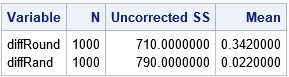The output from PROC MEANS shows the results of generating 1,000 random integers from X. The uncorrected sum of squares (USS) column shows the sum of the squared residuals for each estimator. (The MSE estimate is USS / 1000 for these data.) The table shows that the USS (and MSE) for the rounding estimator is smaller than for the random estimator. On the other hand, The mean of the residuals is not close to zero for the rounding method because it is a biased method. In contrast, the mean of the residuals for the random method, which is unbiased, is close to zero.

It might be easier to see the bias of the estimators if you look at the predicted values themselves, rather than at the residuals. The following call to PROC MEANS computes the sample mean for X and the two methods of predicting X:

/* the rounding method is biased; the random guess is unbiased */ proc means data=Guess n mean stddev; var x PredRound PredRand; run;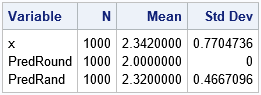This output shows that the simulated values of X have a sample mean of 2.34, which is close to the expected value. In contrast, the rounding method always predicts 2, so the sample mean for that column is exactly 2.0. The sample mean for the unbiased random method is 2.32, which is close to the expected value.

In summary, you can use SAS to simulate a simple example that compares two methods of predicting the value of a discrete random process. One method is biased but has the lowest MSE. The other is unbiased but has a larger MSE. In statistics and machine learning, practitioners often choose between an unbiased method (such as ordinary least squares regression) and a biased method (such as ridge regression or LASSO regression). The example in this article provides a very simple situation that you can use to think about these issues.

The post Predict a random integer: The tradeoff between bias and variance appeared first on The DO Loop.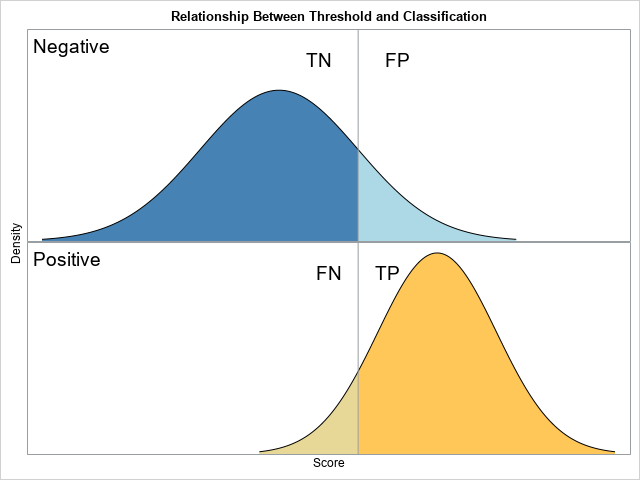The ROC curve is a graphical method that summarizes how well a binary classifier can discriminate between two populations, often called the "negative" population (individuals who do not have a disease or characteristic) and the "positive" population (individuals who do have it). As shown in a previous article, there is a theoretical model, called the binormal model, that describes the fundamental features in binary classification. The model assumes a set of scores that are normally distributed for each population, and the mean of the scores for the negative population is less than the mean of scores for the positive population. The figure to the right (which was discussed in the previous article) shows a threshold value (the vertical line) that a researcher can use to classify an individual as belonging to the positive or negative population, according to whether his score is greater than or less than the threshold, respectively.

In most applications, any reasonable choice of the threshold will misclassify some individuals. Members of the negative population can be misclassified, which results in a false positive (FP). Members of the positive population can be misclassified, which results in a false negative (FP). Correctly classified individuals are true negatives (TN) and true positives (TP).

### Vizualize the binary classification method

One way to assess the predictive accuracy of the classifier is to use the proportions of the populations that are classified correctly or are misclassified. Because the total area under a normal curve is 1, the threshold parameter divides the area into two proportions. It is instructive to look at how the proportions change as the threshold value ranges. The proportions are usually called "rates." The four regions correspond to the True Negative Rate (TNR), False Positive Rate (FPR), False Negative Rate (FNR), and True Positive Rate (TPR).

For the binormal model, you can use the standard deviations of the populations to choose a suitable range for the threshold parameter. The following SAS DATA step uses the normal cumulative distribution function (CDF) to compute the proportion of each population that lies to the left and to the right of the threshold parameter for a range of values. These proportions are then plotted against the threshold parameters.

%let mu_N = 0; /* mean of Negative population */ %let sigma_N = 1; /* std dev of Negative population */ %let mu_P = 2; /* mean of Positive population */ %let sigma_P = 0.75; /* std dev of Positive population */   /* TNR = True Negative Rate (TNR) = area to the left of the threshold for the Negative pop FPR = False Positive Rate (FPR) = area to the right of the threshold for the Negative pop FNR = False Negative Rate (FNR) = area to the left of the threshold for the Positive pop TPR = True Positive Rate (TPR) = area to the right of the threshold for the Positive pop */ data ClassRates; do t = -3 to 4 by 0.1; /* threshold cutoff value (could use mean +/- 3*StdDev) */ TNR = cdf("Normal", t, &mu_N, &sigma_N); FPR = 1 - TNR; FNR = cdf("Normal", t, &mu_P, &sigma_P); TPR = 1 - FNR; output; end; run;   title "Classification Rates as a Function of the Threshold"; %macro opt(lab); name="&lab" legendlabel="&lab" lineattrs=(thickness=3); %mend; proc sgplot data=ClassRates; series x=t y=TNR / %opt(TNR); series x=t y=FPR / %opt(FPR); series x=t y=FNR / %opt(FNR); series x=t y=TPR / %opt(TPR); keylegend "TNR" "FNR" / position=NE location=inside across=1; keylegend "TPR" "FPR" / position=SE location=inside across=1; xaxis offsetmax=0.2 label="Threshold"; yaxis label="Classification Rates"; run;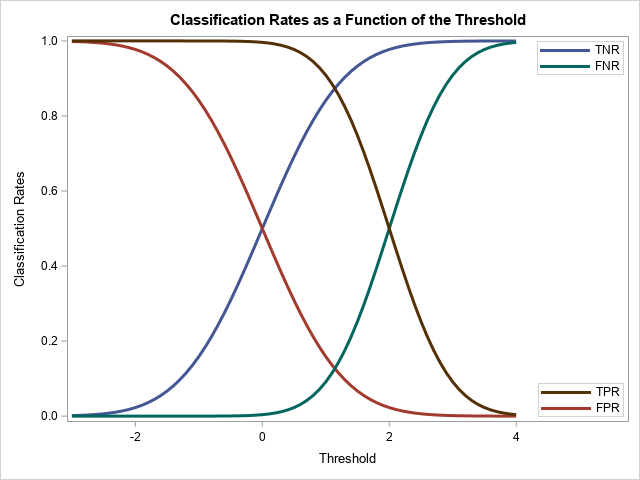The graph shows how the classification and misclassification rates vary as you change the threshold parameter. A few facts are evident:

• Two of the curves are redundant because FPR = 1 – TNR and TPR = 1 – FNR. Thus, it suffices to plot only two curves. A common choice is to display only the FPR and TPR curves.
• When the threshold parameter is much less than the population means, essentially all individuals are predicted to belong to the positive population. Thus, the FPR and the TPR are both essentially 1.
• As the parameter increases, both rates decrease monotonically.
• When the threshold parameter is much greater than the population means, essentially no individuals are predicted to belong to the positive population. Thus, the FPR and TPR are both essentially 0.

### The ROC curve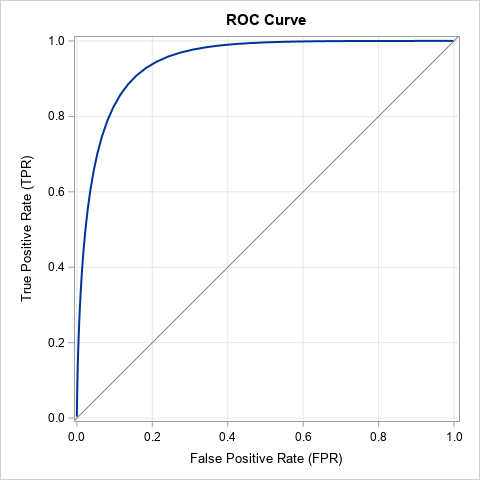The graph in the previous section shows the FPR and TPR as functions of t, the threshold parameter. Alternatively, you can plot the parametric curve ROC(t) = (FPR(t), TPR(t)), for t ∈ (-∞, ∞). Because the FPR and TPR quantities are proportions, the curve (called the ROC curve) is always contained in the unit square [0, 1] x [0, 1]. As discussed previously, as the parameter t → -∞, the curve ROC(t) → (1, 1). As the parameter t → ∞, the curve ROC(t) → (0, 0). The main advantage of the ROC curve is that the ROC curve is independent of the scale of the population scores. In fact, the standard ROC curve does not display the threshold parameter. This means that you can compare the ROC curves from different models that might use different scores to classify the negative and positive populations.

The following call to PROC SGPLOT creates an ROC curve for the binormal model by plotting the TPR (on the vertical axis) against the FPR (on the horizontal axis). The resulting ROC curve is shown to the right.

title "ROC Curve"; title2; proc sgplot data=ClassRates aspect=1 noautolegend; series x=FPR y=TPR / lineattrs=(thickness=2); lineparm x=0 y=0 slope=1 / lineattrs=(color=gray); xaxis grid; yaxis grid; run;

The standard ROC curve does not display the values of the threshold parameter. However, for instructional purposes, it can be enlightening to plot the values of a few selected threshold parameters. An example is shown in the following ROC curve. Displaying the ROC curve this way emphasizes that each point on the ROC curve corresponds to a different threshold parameter. For example, when t=1, the cutoff parameter is 1 and the classification is accomplished by using the vertical line and binormal populations that are shown at the beginning of this article.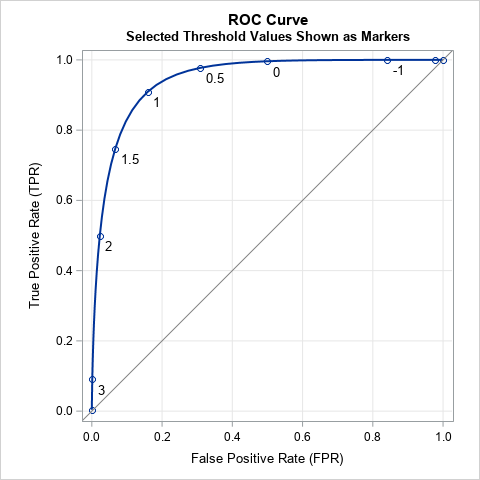### Interpretation of the ROC curve

The ROC curve shows the tradeoff between correctly classifying those who have a disease/condition and those who do not. For concreteness, suppose you are trying to classify people who have cancer based on medical tests. Wherever you place the threshold cutoff, you will make two kinds of errors: You will not identify some people who actually have cancer and you will mistakenly tell other people that they have cancer when, in fact, they do not. The first error is very bad; the second error is also bad but not life-threatening. Consider three choices for the threshold parameter in the binormal model:

• If you use the threshold value t=2, the previous ROC curve indicates that about half of those who have cancer are correctly classified (TPR=0.5) while misclassifying very few people who do not have cancer (FPR=0.02). This value of the threshold is probably not optimal because the test only identifies half of those individuals who actually have cancer.
• If you use t=1, the ROC curve indicates that about 91% of those who have cancer are correctly classified (TPR=0.91) while misclassifying about 16% of those who do not have cancer (FPR=0.16). This value of the threshold seems more reasonable because it detects most cancers while not alarming too many people who do not have cancer.
• As you decrease the threshold parameter, the detection rate only increases slightly, but the proportion of false positives increases rapidly. If you use t=0, the classifier identifies 99.6% of the people who have cancer, but it also mistakenly tells 50% of the non-cancer patients that they have cancer.

In general, the ROC curve helps researchers to understand the trade-offs and costs associated with false positive and false negatives.

### Concluding remarks

In summary, the binormal ROC curve illustrates fundamental features of the binary classification problem. Typically, you use a statistical model to generate scores for the negative and positive populations. The binormal model assumes that the scores are normally distributed and that the mean of the negative scores is less than the mean of the positive scores. With that assumption, it is easy to use the normal CDF function to compute the FPR and TPR for any value of a threshold parameter. You can graph the FPR and TPR as functions of the threshold parameter, or you can create an ROC curve, which is a parametric curve that displays both rates as the parameter varies.

The binormal model is a useful theoretical model and is more applicable than you might think. If the variables in the classification problem are multivariate normal, then any linear classifier results in normally distributed scores. In addition, Krzandowski and Hand (2009, p. 34-35), state that the ROC curve is unchanged by any monotonic increasing transformation of scores, which means that the binormal model applies to any set of scores that can be transformed to normality. This is a large set, indeed, since it includes the complete Johnson system of distributions.

In practice, we do not know the distribution of scores for the population. Instead, we have to estimate the FPR and TPR by using collected data. PROC LOGISTIC in SAS can estimate an ROC curve for data by using a logistic regression classifier. Furthermore, PROC LOGISTIC can automatically create an empirical ROC curve from any set of paired observed and predicted values.

The post The binormal model for ROC curves appeared first on The DO Loop.